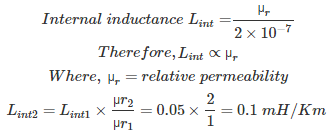# MCQs on Power Systems

##### Page 6 of 67. Go to page 1 2 3 4 5 6 7 8 9 10 11 12 13 14 15 16 17 18 19 20 21 22 23 24 25 26 27 28 29 30 31 32 33 34 35 36 37 38 39 40 41 42 43 44 45 46 47 48 49 50 51 52 53 54 55 56 57 58 59 60 61 62 63 64 65 66 67
01․ In load flow studies PV bus is treated as PQ bus when
phase angle become high
voltage at the bus become high
reactive power goes beyond limit
any of the above

When a network containing one slack bus, one PV bus and the reactive power value of PV bus is in the out of range for a given value of reactive power limits, so that PV bus is working as PQ bus with the value of Q. For the PQ bus the value of Q is the nearest value from the calculated value of Q in the given range.

02․ For accurate load flow calculations on large power systems, the best method is
N-R method
G-S method
Decoupled method
all of the above

Advantages of N-R method: 1. Number of iterations are less, so that it has fast convergence. 2. Convergence is not effected by the choice of slack bus. 3. No need of accelerating factor. 4. Applicable for large power system network. Disadvantages of N-R method: 1. Time taken for each iteration is larger if size of the Jacobian matrix is larger. 2. Computer memory required is larger. 3. Computer programming is difficult.

03․ Find the number of strands of ACSR conductor for 4 layer transmission line?
1
7
37
29

Total number of strands N = 3x² - 3x + 1 Where, x = Number of layers = 4 Total number of strands N = 3×4² - 3×4 + 1 = 37

04․ A 3 layer and diameter of each strand is d, then find the total diameter of ACSR conductor?
d
2d
3d
5d

Total diameter of ACSR conductor D = (2x - 1)×d Where, x = layer number d = diameter of each strand Therefore, Total diameter of ACSR conductor D = (2×3 - 1)×d = 5d

05․ Find the number of strands of ACSR conductor for 3 layer transmission line?
1
7
19
29

Total number of strands N = 3x² - 3x + 1 Where, x = Number of layers = 3 Total number of strands N = 3×3² - 3×3 + 1 = 19

06․ The total number of strands in a ACSR conductor are 7, then find the layer number?
2
3
5
7

Total number of strands N = 3x² - 3x + 1 Here N = 7 Where, x = Number of layers Therefore, 7 = 3x² - 3x + 1 3x² - 3x = 6 3x (x-1) = 6 x (x-1) = 2 ∴ x = 2

07․ An ACSR conductor has a diameter of 2 cm has internal inductance of 0.05 mH/Km. If this ACSR is replaced with another having a diameter of 4 cm then its internal inductance is
0.1 mH/Km
0.025 mH/Km
0.05 mH/Km
0 mH/Km

Internal inductance Lint = µr/2*10-7 Therefore, Lint ∝ µr Where, µr = relative permeability Internal inductance is independent of size. It depends only on relative permeability.

08․ An ACSR conductor has internal inductance 0.05 mH/Km for µr = 1. If this ACSR is replaced with another having µr = 2, then find the internal inductance?
0.1 mH/Km
0.025 mH/Km
0.05 mH/Km
0 mH/Km09․ Telecommunication lines are transposed to reduce the
efficiency
voltage level
all of the above

If power line and telecommunication lines are running close to each other, the current flowing in the power line produces magnetic flux linkage with the communication line conductor induces an emf in the telecommunication line conductor.This is called electro magnetic induction. Similarly due to earth effect electric field is produced by the charges of the earth induces a voltage in between the conductors of the telecommunication lines. This is called electro static induction. This both electro magnetic induction and electro static induction produces the voltage between the telecommunication line conductors which causes interference to the telecommunication signals which is called radio interference. To reduce this radio interference in the telecommunication line, either power line or both power and telecommunication lines are transposed at regular intervals of length the transmission line.

10․ Which of the following produces the radio interference in communication lines?
electro magnetic induction
electro static induction
both 1 and 2
none of the above

If power line and telecommunication lines are running close to each other, the current flowing in the power line produces magnetic flux linkage with the communication line conductor induces an emf in the telecommunication line conductor.This is called electro magnetic induction. Similarly due to earth effect electric field is produced by the charges of the earth induces a voltage in between the conductors of the telecommunication lines. This is called electro static induction. This both electro magnetic induction and electro static induction produces the voltage between the telecommunication line conductors which causes interference to the telecommunication signals which is called radio interference.

<<<45678>>>